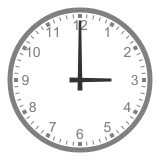# Third Term Examination Mathematics Primary 2 (Basic 2) – Exam Questions

Last Updated on May 25, 2021 by Alabi M. S.

MATHEMATICS

THIRD TERM EXAMINATION

PRIMARY 2 (BASIC 2)

SECTION A

Attempt all the questions.

Choose the correct answer from the options.

1. Money can be used for ____________.

[a] fight

[b] payment for goods and service

[c] none of the above

2. Who picture is on hundred hundred naira note?

[a] Chief Obafemi Awolowo

[b] Alhaji Musa Yar’’adura

[c] Dr Nnamadi Azikwe

3. The highest Nigeria Naira note is ____________.

[a] ₦200

[b] ₦500

[c] ₦1000

4. 60 seconds make ____________.

[a] 2 minute

[b] 4 minutes

[c] 1 minutes

5. A. M.   means ____________.

[a] evening

[b] morning

[c] afternoon

6. P. M. means ____________.

[a] morning

[b] evening

[c] afternoon

7. 60 minutes make ____________.

[a] 2 hours

[b] 3 hours

[c] 1 hour

8. ____________ is the first day of the month.

[a] Sunday

[b] Monday

[c] Friday

9. How many days are there in a week?

[a] 2 days

[b] 8 days

[c] 7 days

10. Add up this 2/6 + 3/6

[a] 7/6

[b] 1/6

[c] 5/6

11. Change the money to Naira: 200k = ____________.

[a] ₦4

[b] ₦2

[c] ₦3

12. What is the product of 40 and 5?

[a] 150

[b] 260

[c] 200

13. What is the shaded part of these Fraction?

[a] 1/6

[b] 1/9

[c] 1/4

14. The odd number is ____________.

[a] 40

[b] 41

[c] 42

15. Add up 347 and 227.

[a] 629

[b] 574

[c] 326

16. The instrument used for measurement length is ____________.

[a] ruler

[b] tape rule

[c] meter rule

17. Tick the longest of the lengths presented below:

[a] 4 m, 100 cm

[b] 50 cm, 1 m

[c] 1 m 1 cm

18. What is the standard unit for measuring objects found in the class?

[a] km and m

[b] m and cm

[c] mm and mc

What says the time?19.3

[a] 1 o’ clock 20

[b] 8 o’ clock

[c] 4 o’  clock20.

[a] half past 2

[b] half past 1

[c] half past 3

Get more Mathematics Exam Questions – Third Term Examination Mathematics Link

SECTION B

Attempt all questions in this section.

QUESTION 1

Find out and tick the area which is smaller:

A. 4 cm X 3 cm = _________ or 5 cm X 4 cm = ________

B. 5 cm X 2 cm = _________ or 2 cm X 4 cm = ________

C. 6 cm X 3 cm = _________ or 5cm X 4 cm = ________

QUESTION 2

Use the cube and cuboid to answer question A – E.

A. How many flat faces does a cube have?

B. How many flat faces does a cuboid have?

C. How many edges does a cube have?

D. How many corners does a cube?

E. What shape forms a cuboid?

QUESTION 3

Answer questions A – E with the aid of the diagram of the sphere and cylinder.

A. A cylinder has ______________ flat surface.

B. Does a sphere have a flat surface?

C. How many corners does a cylinder have?

D. How many curved edges does a cylinder have?

E. What kind of face does a cylinder have?

4. Draw the following shapes.

A. CIRCLE

B. RECTANGLE

C. CUBOID

Follow the link for Mathematics Lessons – Third Term Mathematics Plan Lesson Notes Link

Smart Teachers Plan Lesson Notes - ClassRoomNotes support teachers with hands-on lesson plans/notes, printable and thoughtful teaching resources. @ClassRoomNotes - We always love to hear from you always. Stay connected with your classroom.
error: